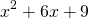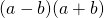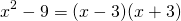# Prime Factors

Spot a square number or a cube number using prime factors

The prime factors of 144 are:These factors can be grouped as:In other words:Which confirms that 144 is a square number.

Your turn – use prime factors to create a square number. Check it out using thekey on your calculator!

Extend the principle to cube numbers!

# Multiplying Polynomials

Multiply any degree by any degree Expanding brackets for polynomials in general

Multiply several brackets Expanding brackets more than two

Kuta software worksheet: Multiplying polynomials (see page 2)

# Problem Solving

Geometry problems – what do you notice? Geometry Problems with Quadratic Expressions notice

Geometry problems – questions attached Geometry Problems with Quadratic Expressions

# Factoring

Spot a trinomial that is a perfect squareFor example:These perfect squares factor as follows:Choose your own values of a, b, and create some of your own.

Spot a trinomial that is the difference of two squares

Any trinomial of the form:is called the difference of two squares. You can confirm that it factors as:For example:These differences of two squares factor as follows:Kuta Software worksheet: special cases

Factor a trinomial where. Read the link to find the various approaches to a trinomial of the form:Kuta Software worksheet: Trinomial with leading coefficient not 1

Two more:

sum of two cubesdifference of two cubes